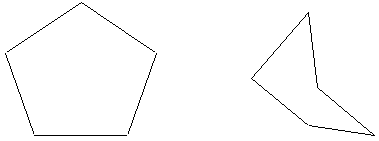# SAT / ACT Prep Online Guides and TipsWondering, “How many sides does a pentagon have?” Look no further! This article will tell you everything you need to know about pentagons.

## Pentagon Sides: What Makes a Pentagon

A pentagon is a type of shape called a polygon.

A polygon is a flat, enclosed shape that is made up of straight lines. To be “enclosed’ means that all sides of the polygon connect and none of the sides are curved.

There are two basic categories of polygons: regular and irregular. Regular polygons have equal sides and equal angles. Irregular polygons do not.

Pentagons can be both irregular and regular, meaning that they can have both equal sides and equal angles as well as have unequal sides and unequal angles.## How Many Sides Does a Pentagon Have?

A pentagon is any polygon that has five sides and five angles. The root of the word pentagon is “penta” which comes from the Greek word for five. “Gon” comes from the Greek word for angle. So, if you put the two words together, you get “five angles,” which corresponds to how many angles are in the shape.

As I just mentioned, in order for a shape to qualify as a pentagon, it needs to be a five sided shape and have five angles. The pentagon’s sides need to be straight: if any of them are curved, then the shape does not qualify as a pentagon.

A regular pentagon has all equal sides and angles. In a regular pentagon, its interior angles are 108 degrees and its exterior angles are 72 degrees. The angles of a pentagon add up to 540 degrees.

In an irregular pentagon, pentagon sides and angles can be different sizes.The shape on the left is a regular pentagon. It has five sides and five angles, all of which are the same length or size.

The shape on the right is an irregular pentagon. Each side is a different length. Each angle is a different size.

## Other Types of Polygons

Pentagons are a 5 sided shape. Other polygons have different amounts of sides and angles.

Here’s a list of other polygons and the number of sides they have.

 Polygon Number of Sides Triangle 3 Quadrilateral 4 Pentagon 5 Hexagon 6 Heptagon 7 Octagon 8 Nonagon 9 Decagon 10 Hendecagon 11 Dodecagon 12 Tridecagon 13 Tetradecagon 14 Pentadecagon 15 Hexadecagon 16 Heptadecagon 17 Octadecagon 18 Nonadecagon 19 Icosagon 20 Triacontagon 30 Tetracontagon 40 Pentacontagon 50

## Pentagon Sides: Final Thoughts

A pentagon is a type of 5 sided shape called a polygon. Polygons are flat, enclosed shapes with sides that are straight lines. How many sides does a pentagon have? A pentagon is a polygon that has five sides.

## What's Next?

Make sure you’re prepared for the SAT and ACT math tests by reading our Ultimate Guide to SAT Math Prep and our Ultimate Guide to ACT Math Prep!

If you’re feeling pretty confident, why not test your skills against the 13 hardest SAT Math questions ever? If you can conquer those, you’ll likely do great on the SAT, too! (Here are the hardest math questions on the ACT, too.)

If you need a little extra practice, why not check out some math prep books? Here’s a list of our favorites.